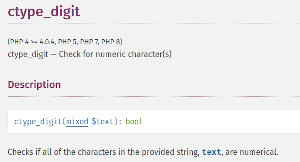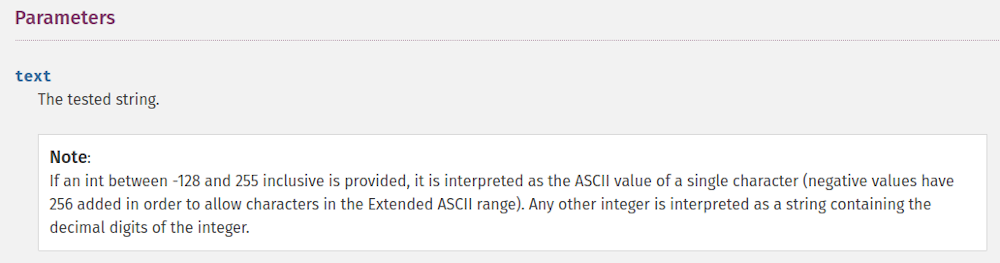# Security Issues: ctype_digit() WeirdnessIf you've ever attended a PHP course, when considering adding form data validation, most instructors will follow the conventional wisdom, which says to avoid using regular expressions if you are able to use any of the PHP ctype_*() family of functions instead.  This handy set of functions is indeed convenient, fast, and have all been in the language for ages.  In contrast with the is_*() functions, instead of determining the data type of a variable, the ctype_*() family examines the contents of the variable.  There is a little bit of weirdness surrounding ctype_digit(), however, of which you might not be aware.  In this article we examine this function's dirty little secret and show you how to avoid ending up with false positives when performing form data validation.

Probably a good starting point when discussing ctype_digit() is to have a look at it's signature.  Consulting the documentation page, we come up with this:What's important about this signature is that the data type of the variable to be examined is mixed.  This means that, at least theoretically, ctype_digit() is able to examine any variable and return TRUE or FALSE depending on whether or not its contents are 0 - 9 only.

## Using ctype_digit() for Form Data Validation

In the following example, let's assume that you're validating form data.  For the sake of this example, let's assume that \$_POST contains the following:

``````\$_POST = [
'id' => '1111',
'age' => '49',
'gender' => 'M',
'amount' => '99.99',
'life_universe_and_everything' => '42'
];``````

If we then run the post data through a foreach() loop, and validate using ctype_digit():

``````\$ptn = "%30s : %s\n";
printf(\$ptn, 'Form Field', 'Only Digits');
foreach (\$_POST as \$key => \$value)
printf(\$ptn, \$key, (ctype_digit(\$value) ? 'Y' : 'N'));``````

The resulting output would appear as follows:

``````                    Form Field : Only Digits
id : Y
age : Y
gender : N
amount : N
life_universe_and_everything : Y
``````

OK, so far so good.  So ... what's the problem?

## Houston We Have a Problem!

What a developer might next do with the data, prior to validation, is perform a normal bit of sanitization.  Here's how the sanitizing code might appear:

``````\$id = \$_POST['id'] ?? 0;
\$age = \$_POST['age'] ?? 0;
\$gender = \$_POST['gender'] ?? '';
\$amount = \$_POST['amount'] ?? 0.00;
\$life_etc = \$_POST['life_universe_and_everything'] ?? 0;

\$_POST['id'] = (int) \$id;
\$_POST['age'] = (int) \$age;
\$_POST['gender'] = (in_array(\$gender, ['M','F','X'])) ? \$gender : 'X';
\$_POST['amount'] = (float) \$amount;
\$_POST['life_universe_and_everything'] = (int) \$life_etc;``````

However, if we then run the sanitized code through the same loop as shown above, the result is slightly different, as seen here:

``````                    Form Field : Only Digits
id : Y
age : Y
gender : N
amount : N
life_universe_and_everything : N
``````

The last item should come back as containing only digits, as it's the number 42.  But, as you can see from the output, we now have a false positive.  Help!!!  What's going on?

## ctype_digit's Dirty Little Secret

The answer to this perplexing problem can be found if we return the the PHP documentation page for ctype_digit().As you can see from the documentation, although ctype_digit() accepts arguments of mixed data type, if the data type is `int`, and the value is between -128 and 255, it's treated as an ASCII code!  So, in the example shown just above, the `id` field, with a value of 1111, passed without a problem.  The `life_universe_and_everything` field, on the other hand, was treated as an ASCII character 42, which happens to be the code for an asterisk (*).  Since an asterisk is not a digit, the return value from ctype_digit() was FALSE.

## Got It ... But What About Age?

But now we have a bigger problem: why did `age` return TRUE?  The answer to this again takes us back to the ASCII table.  The value for `age` was 49.  A look at the ASCII table shows us that 49 is the ASCII code for the number one!  Accordingly ctype_digit() returns a value of TRUE, as one is certainly a digit.

## Final Thoughts

When performing form data validation using any of the ctype_*() family of functions, especially ctype_digit(), it would be best to leave the data present in `\$_POST` (or `\$_GET`) in its original state while performing validation.  The ctype_*() family works best with string data, despite the fact that it technically accepts any data type.  You can then perform sanitization after the validation has occurred.